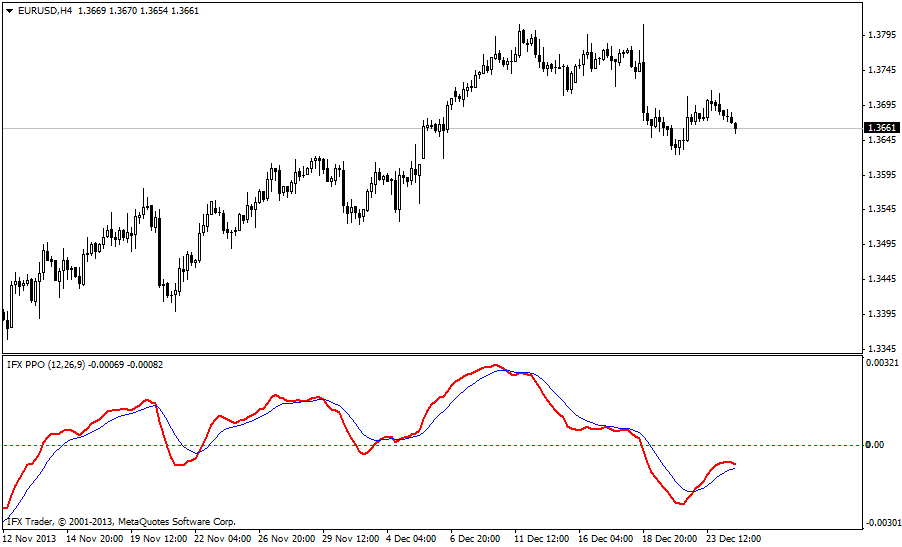www.instaforex.eu >
a website operated by

# PPO Indicator

PPO is a technical momentum indicator measuring the difference between the 26-day and the 9-day exponential moving averages. The indicator is similar to the moving average convergence divergence (MACD) in terms of both practical use and efficiency.

#### Formula

PPO = (EMA(12) – EMA(26) / EMA(26)) x 100

The major difference between the PPO and the MACD is in the concept that the MACD measures the absolute difference between two moving averages, while the PPO calculates the relative difference, in percentage terms.

If fast moving average (red line) is higher than slow moving average (blue line), then uptrend prevails on the market. If fast moving average is lower than slow moving average, then the downtrend prevails on the market. The point of crossing of two moving averages is the point of market entry in the direction of this crossing.

The relevant peculiarity of the indicator, which should be taken into account, is the crossing of signal line of the zero level. If the crossing occurs from the bottom up, then the stable trend is observed, and consequently, one should either open buys, or continue holding it. If the zero line crosses below the signal MA, one should operate with downward trend.

As well as the MACD, the PPO points the situations of divergence and convergence of the indicator with the price movement. If the divergence is observed, it suggests quick price reversal downwards. Meanwhile, if the convergence is observed amid the drop of the price, it is a relevant indicator suggesting forthcoming price reversal upwards.You are now leaving www.instaforex.eu, a website operated by INSTANT TRADING EU LTD
Can't speak right now?